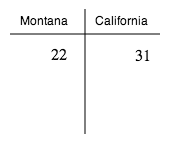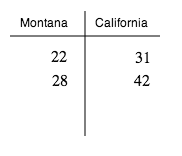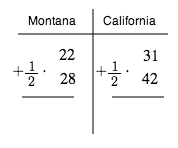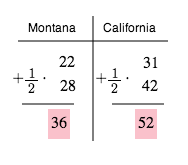### Home > CAAC > Chapter 1 > Lesson 1.1.3 > Problem1-29

1-29.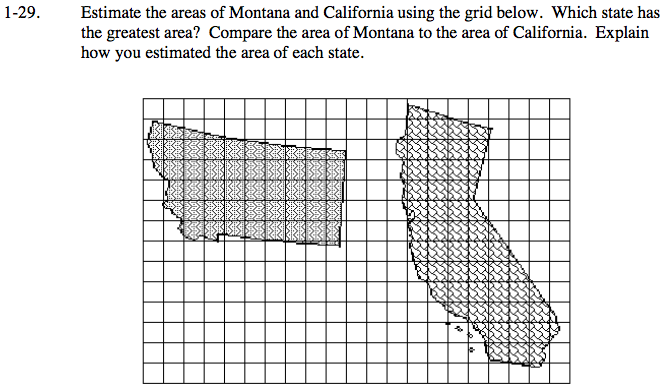Find the number of whole squares each state contains.

Find the number of partial squares each state contains.

Assume that the partial squares have an average area of half a square. Use this assumption to estimate the area of each state.